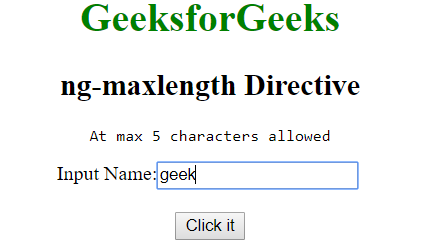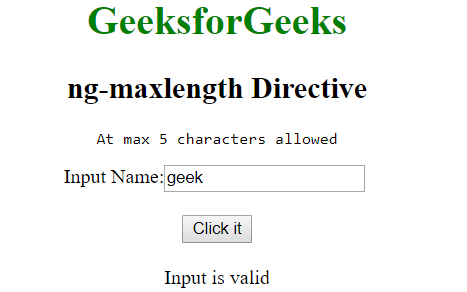# AngularJS | ng-maxlength Directive

• Last Updated : 26 Mar, 2019

The ng-maxlength Directive in AngularJS is used to set the maximum length for an input field i.e it adds the restriction for an input field. It is different from maxlength attribute in HTML because the former prevent users from exceeding the limit whereas the later doesn’t do that. It will make the form invalid if the input limit exceeds.

Syntax:

` <element ng-maxlength="expression"> Contents... </element> `

Where expression is a number denoting the maximum limit upto which the input is valid.

Example: This example uses ng-maxlength Directive to check the maximum length of string.

 ```<``html``>`` ` `<``head``>``    ``<``title``>ng-maxlength Directive``     ` `    ``<``script` `src``=``"https://ajax.googleapis.com/ajax/libs/angularjs/1.6.9/angular.min.js"``>``    `````` ` `<``body` `style``=``"text-align: center"``>``     ` `    ``<``h1` `style``=``"color:green"``>GeeksforGeeks``     ` `    ``<``h2``>ng-maxlength Directive``     ` `    ``<``div` `ng-app``=``"app"` `ng-controller``=``"geek"``>``          ``<``form` `name``=``"maxlen"` `novalidate ``ng-submit``=``                        ``"maxlen.\$valid &&maxlength()"``>``              ``<``pre``>Atmax 5 characters required``               ` `              ``Input: <``input` `type``=``"text"` `name``=``"code"` `ng-model``=``"txt"``                        ``ng-maxlength``=``"5"` `required />``             ``<``br``><``br``>``              ` `             ``<``button` `type``=``"submit"``>Click it``              ` `             ``<``br``><``br``>``              ` `             ``<``span``>{{msg}}``         ````    ````     ` `    ``<``script``>``        ``var app = angular.module('app', []);``        ``app.controller('geek', function (\$scope) {``            ``\$scope.maxlength = function () {``                ``\$scope.msg = "Input is valid";``            ``};``        ``});``    `````` ` ``

Output:
Before clicking the button:After clicking the button:My Personal Notes arrow_drop_up